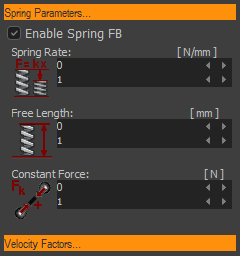﻿ MechDesigner Reference & User Interface > Dialog-Boxes > Dialog: Function-Block: Spring

# Dialog: Function-Block: Spring

Navigation:  MechDesigner Reference & User Interface > Dialog-Boxes >

# Dialog: Function-Block: Spring

## Spring FB

Use a Spring FB to apply a force between two Points. The name we use for the two Points is Anchor-Points.

Machine Settings > Engineering Units

Configure Power Source

### Edit the Spring FBIn Mechanism-Editor:

 1.Double-click the Spring-FB in the graphic-area- or - 1.

### Spring dialog-boxSpring Parameters☑ Check-box to enable the Spring FB.Spring-Rate: Spring-Force / linear unit difference (SI: N/mm) that the Anchor-Points deviate from the Free-Length. (see Force Units and Linear Units). Free-Length: Is the natural-length of the Spring, as it would be if it were not joined to the Anchor-Points. If the distance between Anchor-Points > Free-Length, then the Force-Vector (due to the Spring-Rate only) will Pull Anchor-Points together (o>>>|<<>>o) If the distance between Anchor-Points = Free-Length, then the Force-Vector (due to the Spring-Rate only) = 0 Constant-Force: A positive force will PULL Anchor-Points together (o>>> + <<>>o)

Velocity ParametersCoulomb Friction Force: (Ff = μ.Fn ) A Constant Force that is opposite to the direction of the motion. This parameter is intended to be similar to a Friction Force. However, it is NOT exactly Friction Force, because this force is not a function of the 'Normal Force'. Note: Friction Force is not a Kinetostatic Force. Viscous Coefficient: (Fv α V ( N/(mm/s) ) A Force that is proportional to the relative velocity between the Points. The Force is opposite to the direction of motion. E.g.: If the Damping Coefficient = 4 and the Velocity is +100mm/s, (anchor points moving away from each other’), then force pulling the points together is a force of 400N. Drag Factor: (Fd α V2) ( N/((mm/s)2 ) ) A Force that is proportional to square of the relative velocity between the Points. For example, air resistance. The Force is opposite to the direction of motion. E.g. If the Drag Factor = 4, and the Velocity = +100mm/s (anchor-points moving away from each other’), then force pulling the points together is a force of 40000N.

#### Input-Connector and Output-Connectors

Note: It is not necessary to connect a wire to its input-connector or from its output-connectors. The connectors provide extra functionality.

 Input-Connector Connect a wire to the input-connector to add a force-function between the Spring's Anchor-Points. The units of the data at the input-connector must be Newtons. Use a Maths FB to convert the data-type of a function to the Force data-type. For example, the function might be designed as a motion. In which case, you would need to connect the output of a Motion FB , which has units of mm or degrees to a Math FB to convert the units to Newtons. Note 1: Units inside the Maths FB become SI units. To convert 100mm at the input to a Maths FB to 100N, you must: 1.Change the Output Data Type to Force (N)AND 2.Multiply the input-value by 1000 to convert 0.1m to 100NNote 2 To be consistent with the 'Constant-Force' Parameter: •A positive force at the input will tend to PULL Anchor-Points together (o>>> + <<>>o)TOP output-connector Dimension, Velocity and Acceleration relating to the distance between the Spring's anchor-points. Bottom output-connector Total Applied-Force between the Spring's anchor-points. oTotal Applied-Force = Force at the Input-connector + Force that results from the parameters as set in the Spring FB dialog-box or oDriving-Force = Linear Motive Force, if you configure the Spring FB as a Linear Motor -